## Understanding the Hilbert curve

The Hilbert curve fills space with good properties for sorting N-dimensional data in a linear fashion. I present an IPython notebook with the complete code to follow the algorithm of C. Hamilton CS-2006-07.

Update on 12 june 2015: addition of compact Hilbert indices.

In :
%matplotlib inline
import matplotlib
import matplotlib.pyplot as plt
import numpy as np
import math
from mpl_toolkits.mplot3d import Axes3D
from __future__ import print_function, division


# Understanding the Hilbert curve¶

This notebook is originally developed in a github repository and presented on my blog. The code is 3-clause BSD and content CC-BY.

In this notebook, I review the algorithm by C. H. Hamilton to compute Hilbert curves and indices. The Hilbert curve is a space-filling curve that has good data locality to map N-dimensional data onto a linear space. It is based on a basis curve that visits the $2^N$ vertices of the N-dimensional binary space and that is replicated recursively $M$ times to map $2^{N M}$ points. The curve is the series of points in N-dimensional space and the index of a point is its place on this curve, computing it is subtle. The algorithm is presented in Compact Hilbert Indices, Dalhousie University Technical Report CS-2006-07, July 2006 (here after [TR]).

My motivations (and yours, maybe) are:

• Understand the algorithm to implement it in a simulation code. The point is to sort particles spatially for better memory access.
• Provide a full Python-based implementation that can be used for reference and illustration purposes.
• Have a clear explanation for later reference.

The notebook is self-contained: it contains all the code for all the intermediate operations defined in [TR]. Hold on for the graphic illustrations, they will come as the algorithm is transformed into code.

## Bitwise operations¶

I review here the bitwise operations in Python, as they will be used throughout. Only integer numbers are used. We define a word length that corresponds to the dimension of the Hilbert curve.

The examples use N=3 so that each single integer in $[0:2^N-1]$ can be mapped to a point in 3 dimension that is one vertex of the unit cube. Bitwise operations act as transformations on the integers and equivalently as geometrical operations in 3 dimensions. The XOR operator represents a reflection with respect to one or several of the symmetry planes of the cube. The rotation operator represents a rotations of a third around the diagonal, i.e. the line joining (0,0,0) and (1,1,1).

The function bin_str helps to visualize bitwise operations by converting an integer to its three bits. The right-most bit represents the x-axis, the middle bit the y-axis and the left-most bit the z-axis. In Python, the AND &, OR | and XOR ^ operators are defined and also the bit shifts (>> to the right, or less significant bits and << to the left or more significant bits). The NOT operator ~ requires further masking: It changes the sign bit of the integer. We wish here to operate on a N-bit space and thus cancel any bit beyond the N less significant bits of the integer. The fact that we remain with N non-zero bits and that NOT is operating properly is checked below. We also need rotation operators (like the shift operators but that transfer the over- and under-flowing bits around the 3-bit space) and a function to extract the i-th bit from a number, bit_component.

In :
N = 3

def bin_str(i):
"""Return a string representation of i with N bits."""
out = ''
for j in range(N-1,-1,-1):
if (i>>j) & 1 == 1:
out += '1'
else:
out += '0'
return out

def rotate_right(x, d):
"""Rotate x by d bits to the right."""
d = d % N
out = x >> d
for i in range(d):
bit = (x & 2**i)>>i
out |= bit << (N+i-d)
return out

def rotate_left(x, d):
"""Rotate x by d bits to the left."""
d = d % N
out = x << d
excess = out
out = out & (2**N-1)
for i in range(d):
bit = (x & 2**(N-1-d+1+i))>> (N-1-d+1+i)
out |= bit << i
return out

def bit_component(x, i):
"""Return i-th bit of x"""
return (x & 2**i) >> i

In :
print('AND  ', bin_str(1), '&', bin_str(5), '=', bin_str(1 & 5))
print('OR   ', bin_str(1), '|', bin_str(5), '=', bin_str(1 | 5))
print('XOR  ', bin_str(1), '^', bin_str(5), '=', bin_str(1 ^ 5))
print('shift', bin_str(2), '>> 1  =', bin_str(2 >> 1))
print('shift', bin_str(2), '<< 1  =', bin_str(2 << 1))
print('rot  ', bin_str(1), '↻', bin_str(rotate_right(1, 1)), '↻',
bin_str(rotate_right(1,2)), '↻', bin_str(rotate_right(1,3)))
print('NOT  ', bin_str(1), '      =', bin_str(~1 & 2**N-1))

# verify that '~i & 2**N-1' performs the NOT operation in N-bit space
for i in range(2**N):
not_i = ~i & 2**N-1
assert not_i >=0
assert not_i < 2**N
assert i & not_i == 0
assert i | not_i == 2**N-1

AND   001 & 101 = 001
OR    001 | 101 = 101
XOR   001 ^ 101 = 100
shift 010 >> 1  = 001
shift 010 << 1  = 100
rot   001 ↻ 100 ↻ 010 ↻ 001
NOT   001       = 110


The technical reports describes with good details all the intermediate steps of the algorithm using binary operations and sequences. The gray code index of a number i, for instance, is given by gc(i) = i XOR (i ≫ 1).

To understand the building of the Hilbert curve, other quantities are needed. The graphical representation use arrows in each sub-hypercube of the curve to represent the path. The definitions of the gray curve index and of the arrows is given and we use these defintions to illustrate the Hilbert curve afterwards.

The interpretation of gc, e and f below is a binary representation in base N of the vertices of a hypercube (a square for N=2, a cube for N=3, etc). The bits represent the z, y and x coordinates.

In :
# Build all edges of a square and of a cube

edges = [((0,),(1,))]

"""Extend the list of edges from an hypercube to the list of
edges of the hypercube of the next dimension."""

old_edges = list(edges)
old_points = set( [x for x in old_edges] ) | set( [x for x in old_edges] )
edges = [ ( (0,)+x, (0,)+x ) for x in old_edges ]
edges.extend( [ ( (1,)+x, (1,)+x ) for x in old_edges ] )
for e in old_points:
edges.append( ( (0,)+e, (1,)+e) )

return edges

cube_xyz = []
for single_edge in cube_edges:
cube_xyz.append(single_edge)
cube_xyz.append(single_edge)
# The np.inf value breaks the line plot into disconnected parts
cube_xyz.append((np.inf,)*3)
cube_xyz = np.array(cube_xyz)

def set_unit_cube(ax, side=1, set_view=(10,-67)):
"""Present the unit cube."""
ax.set_xlabel('x'); ax.set_ylabel('y'); ax.set_zlabel('z');
ax.set_xticks(range(side+1)); ax.set_yticks(range(side+1)); ax.set_zticks(range(side+1));
ax.set_xlim(0, side); ax.set_ylim(0,side); ax.set_zlim(0,side);
if set_view:
ax.view_init(*set_view)

fig = plt.figure(figsize=(6, 5))

ax.plot(*zip(*cube_xyz), color='b')

for i in range(2**N):
x = bit_component(i, 0)
y = bit_component(i, 1)
z = bit_component(i, 2)
ax.text(x, y+0.05, z+0.05, bin_str(i))

set_unit_cube(ax)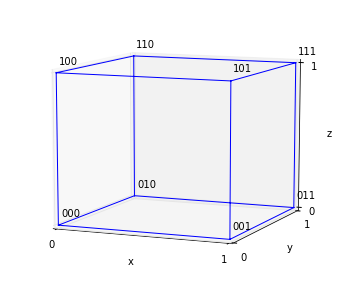In :
def gc(i):
"""Return the Gray code index of i."""
return i ^ (i >> 1)

def e(i):
"""Return the entry point of hypercube i."""
if i==0:
return 0
else:
return gc(2*int(math.floor((i-1)//2)))

def f(i):
"""Return the exit point of hypercube i."""
return e(2**N-1-i) ^ 2**(N-1)

def i_to_p(i):
"""Extract the 3d position from a 3-bit integer."""
return [bit_component(i,j) for j in (0,1,2)]

In :
print('Gray code', map(lambda i: (gc(i), bin_str(gc(i))), range(2**N)),
'Entry point', map(lambda i: (e(i), bin_str(e(i))), range(2**N)),
'Exit point', map(lambda i: (f(i), bin_str(f(i))), range(2**N)),
sep='\n')

Gray code
[(0, '000'), (1, '001'), (3, '011'), (2, '010'), (6, '110'), (7, '111'), (5, '101'), (4, '100')]
Entry point
[(0, '000'), (0, '000'), (0, '000'), (3, '011'), (3, '011'), (6, '110'), (6, '110'), (5, '101')]
Exit point
[(1, '001'), (2, '010'), (2, '010'), (7, '111'), (7, '111'), (4, '100'), (4, '100'), (4, '100')]


## Visiting the Hilbert curve in the cube¶

The path of the Hilbert curve without recursion is encoded in the sequence gc(i), whose 3-bit values are interpreted a points in 3D (see above), and that visits the edges of the cube. These coordinates (scaled by 1/2) are stored in xyz.

The first figure displays the curve visiting the centers of the subcubes, one of the typical representations of the Hilbert curve. In [TR], the path of the Hilbert curve proceeds by entering subcube gc(i) at point e(i) and leaving it a point f(i). The entry and exit points e(i) and f(i) are stored in e_xyz and f_xyz and shown below. The last figure represents the full path as the sequences of arrows joining those points.

In :
# Obtain the 3 d coordinates for gc(i), e(i) and f(i) to build the Hilbert curve
xyz = np.array(map(lambda i: i_to_p(gc(i)), range(2**N)))/2
e_xyz = np.array(map(lambda i: i_to_p(e(i)), range(2**N)))/2
f_xyz = np.array(map(lambda i: i_to_p(f(i)), range(2**N)))/2

fig = plt.figure(figsize=(14, 12))

ax1.plot(*zip(*(xyz+0.25)))
for i, (x,y,z) in enumerate((xyz+0.27)):
ax1.text(x, y, z, str(i))
ax1.set_title('The Hilbert curve, subcube centered')

edges = []
for e_point, f_point, origin in zip(e_xyz, f_xyz, xyz):
dir = (f_point-e_point)
# For the 3d quiver plot, the origin of the arrow is shifted
# which is why we use origin+e_point+dir instead of origin+e_point
edges.append(np.concatenate((origin+e_point+dir, dir)))

ax2.quiver(*zip(*edges), length=0.5)
ax2.set_title('Arrows to visit the Hilbert curve')

# A naive sequence of binary points in 3D
c = 0.25 + np.array(map(i_to_p, range(2**N)))/2

ax3.plot(*zip(*c), color='b')
for i, (x,y,z) in enumerate(c):
ax3.text(x, y, z, str(i))

ax3.set_title('A naive curve to visit the cube')

for ax in [ax1, ax2, ax3]:
ax.plot(*zip(*cube_xyz), color='k', zorder=1, alpha=0.5)
set_unit_cube(ax)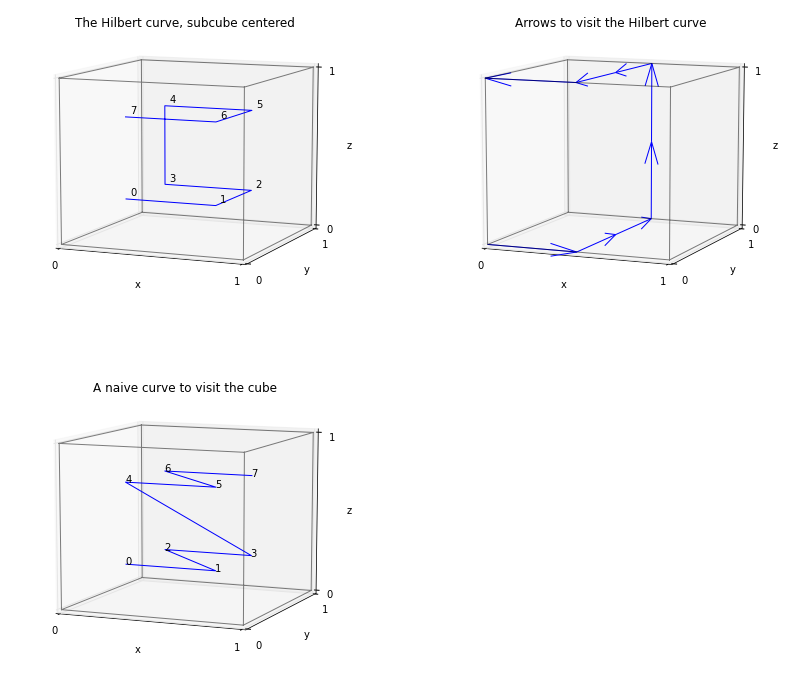## Define the curve directions and transforms¶

To write the Hilbert curve algorithm, we still need to define the inter-subcube direction g and the intra-subcube direction d (the direction of the arrow that connect e and f).

In :
def inverse_gc(g):
"""The inverse gray code."""
i = g
j = 1
while j<N:
i = i ^ (g >> j)
j = j + 1
return i

def g(i):
"""The direction between subcube i and the next one"""
return int(np.log2(gc(i)^gc(i+1)))

def d(i):
"""The direction of the arrow whithin a subcube."""
if i==0:
return 0
elif (i%2)==0:
return g(i-1) % N
else:
return g(i) % N

def T(e, d, b):
"""Transform b."""
out = b ^ e
return rotate_right(out, d+1)

def T_inv(e, d, b):
"""Inverse transform b."""
return T(rotate_right(e, d+1), N-d-2, b)


## Validating the relations¶

The algorithm for the Hilbert curve is based on a series of relations between e, f, d and g. We can check those easily now. In order, we verify that:

• Only the g(i)-th bit is changed between gc(i) and gc(i+1)
• The tranform T sends an entry point e(i) to zero and the exit point f(i) to $2^{N-1}$: $T_{e(i),d(i)}(e(i))=0$ and $T_{e(i),d(i)}(f(i))=f(i)$
• The entry point e(i) reflected along the direction d(i) is the exit point f(i): $e(i)$ ^ $d(i) = f(i)$
• The inverse transform $T^{-1}$ composed with the direct transform $T$ is the identity.
In :
# only g(i)-th bit changes from gc(i) to gc(i+1)
for i in range(2**N-1):
assert gc(i) ^ (1 << g(i)) == gc(i+1)

# T sends e(i) to 0 and f(i) to 2**(N-1)
for i in range(2**N):
assert T(e(i), d(i), e(i))==0
assert T(e(i), d(i), f(i))==2**(N-1)

# e(i) reflected in direction d(i) is f(i)
for i in range(2**N):
assert e(i) ^ (1<<d(i)) == f(i)

# T_inv composed with T (and vice versa) is the identity operator
for i in range(2**N):
for b in range(2**N):
assert T(e(i), d(i), T_inv(e(i), d(i), b)) == b
assert T_inv(e(i), d(i), T(e(i), d(i), b)) == b


## Transform the curve for the subcubes¶

According to [TR], one can use the transform T to map a subcube into the larger cube. Using the inverse transform, it is thus possible to generate the recursed Hilbert curve by mapping the main curve into each subcube. The coordinates of this refined Hilbert curve lie within the subcubes and must be translated by the corresponding origins to fill space correctly.

We construct below the refined Hilbert curve by this manual procedure and display the individual subcubes. The coordinates are then used to show the directed path followed by the curve.

In :
fig = plt.figure(figsize=(12, 18))

# store the Hilbert curve with no recursion
main_curve = [gc(i) for i in range(2**N)]
# list of the in-subcube curves
sub_curves = []
for i, h_idx in enumerate(main_curve):
# append points from the subcube (obtained with the inverse transform T_inv
points = np.array( [ i_to_p(T_inv(e(i), d(i), x)) for x in main_curve ] )
# Translate the points by the origin of the subcube
points += np.array(i_to_p(h_idx))*2
sub_curves.append( points )

# plot all subcurves separately
[ax.plot(*zip(*c)) for c in sub_curves]
ax.set_title('Individual Hilbert "subcubes"')
set_unit_cube(ax, 3,  set_view=False)

# prepare the data to draw arrows with the quiver command
cc = np.concatenate(sub_curves)
U = cc[1:,0]-cc[:-1,0]
X = cc[:-1,0]+U
V = cc[1:,1]-cc[:-1,1]
Y = cc[:-1,1]+V
W = cc[1:,2]-cc[:-1,2]
Z = cc[:-1,2]+W
ax.quiver(X,Y,Z,U,V,W,color=[matplotlib.cm.gnuplot(x) for x in np.linspace(0, 1,len(X)*3)], length=1)
ax.set_title('Connected Hilbert "subcubes"')
set_unit_cube(ax, 3, set_view=False)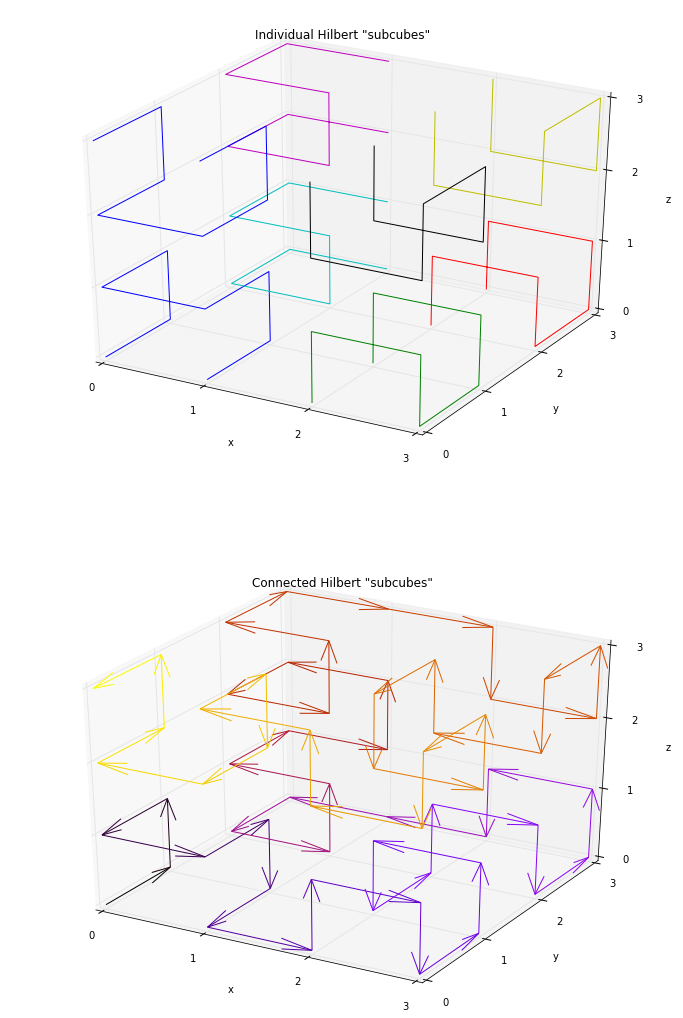## Implementation of the algorithms¶

Now that all the components of the algorithm have been reviewed, it is time for the actual code. In [TR], the algorithm is listed explicitly in pseudo-code.

• Algorithm 2, implemented in TR_algo2, transforms a set of integer coordinates into its Hilbert index.
• Algorithm 3, implemented in TR_algo3, transforms a Hilbert index into a set of coordinates.

Algorithm 2 works by computing the Hilbert index at subsequent refinement levels. Starting from the most significant bits of the coordinates p, it computes the subcube to which p belongs as a label l. l encodes in its N bits the subcube of interest as was show in the unit cube earlier. From l, it is easy to obtain the Hilbert index. The final Hilbert index is composed of this data packed in a single integer variable. As it proceeds in the subcube, the algorithm composes the transform T by updating the current entry point ve direction vd according to Lemma 2.13 of [TR].

Algorithm 3 works in a similar manner but extracts the relevant bits of the Hilbert index at each refinement level to construct the coordinates of the point.

In :
M = 2
def TR_algo2(p):
"""Return the Hilbert index of point p"""
# h will contain the Hilbert index
h = 0
# ve and vd contain the entry point and dimension of the current subcube
# we choose here a main traversal direction N-2 (i.e. z for a cube) to match
# the illustrations
ve = 0
vd = 2
for i in range(M-1, -1, -1):
# the cell label is constructed in two steps
# 1. extract the relevant bits from p
l = [bit_component(px, i) for px in p]
# 2. construct a integer whose bits are given by l
l = sum( [lx*2**j for j, lx in enumerate(l)] )
# transform l into the current subcube
l = T(ve, vd, l)
# obtain the gray code ordering from the label l
w = inverse_gc(l)
# compose (see [TR] lemma 2.13) the transform of ve and vd
# with the data of the subcube
ve = ve ^ (rotate_left(e(w), vd+1))
vd = (vd + d(w) + 1) % N
# move the index to more significant bits and add current value
h = (h << N) | w
return h

def TR_algo3(h):
"""Return the coordinates for the Hilbert index h"""
ve = 0
vd = 2
p = *N
for i in range(M-1, -1, -1):
w = [bit_component(h, i*N+ii) for ii in range(N)]
#print(i, w)
w = sum( [wx*2**j for j, wx in enumerate(w)] )
#print(i, w, gc(w))
l = gc(w)
l = T_inv(ve, vd, l)
for j in range(N):
p[j] += bit_component(l, j) << i
ve = ve ^ rotate_left(e(w), vd+1)
vd = (vd + d(w) + 1) % N
return p


## Displaying and checking the algorithm¶

A basic consistency check is to verify that the successive application of TR_algo3 and TR_algo2 returns the same number than the one given.

A visual inspection of the Hilbert curve on which we superimpose the Hilbert indices confirms the consistency of the algorithm.

In :
# Verifying that the algorithm and its inverse agree
for h_idx in range(2**(N*M)):
assert TR_algo2(TR_algo3(h_idx)) == h_idx

In :
fig = plt.figure(figsize=(12,9))

ax.quiver(X,Y,Z,U,V,W,color=[matplotlib.cm.gnuplot(x) for x in np.linspace(0, 1,len(X)*3)], length=1)
ax.set_title('Hilbert indices in 3D space')

for h in range(2**(N*M)):
x, y, z = TR_algo3(h)
ax.text(x, y, z+0.1, str(h))

set_unit_cube(ax, 3, set_view=(15,-55))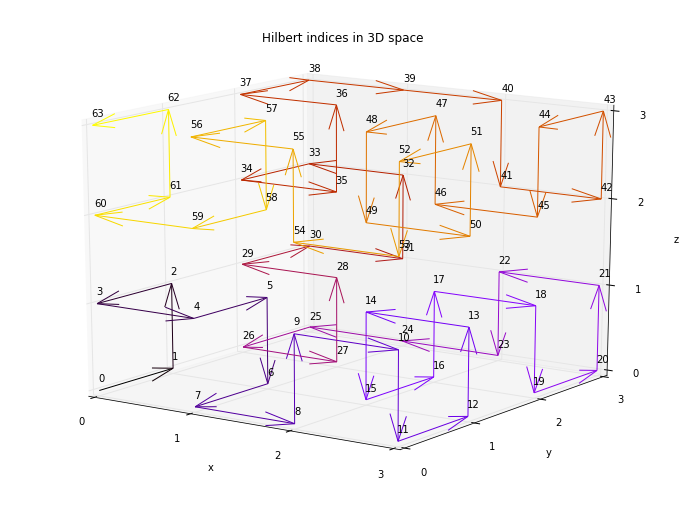## Compact indices¶

One novelty in [TR] is the definition of a compact Hilbert index that enables efficient storage of indices for spaces that have unequal sides. Think of it as allowing non-cubic boxes in 3D.

The ideas underlying this compact index are:

• The ordering of the index with respect to the full Hilbert index should be conserved.
• Some bits will be meaningless to store as they always be equal to zero and as such they will be skipped. In practice, it means that depending on the refinement (or recursion) level, less than N bits may be stored per level.

The algorithms below are very similar to the previous ones, with the addition of masking to retain only meaningful bits in the index. After the implementations, we perform a check that the direct and inverse algorithms agree and illustrate the final result.

In :
def gcr(i, mu, pi):
"""Compute the gray code rank of i given the mask mu.
Algorithm 4 in [TR]"""
r = 0
for k in range(N-1, -1, -1):
if bit_component(mu, k):
r = (r << 1) | bit_component(i, k)
return r

def gcr_inv(r, mu, pi):
"""Inverse of the gray code rank, given the mask mu and the pattern pi.
Algorithm 5 in [TR]"""
i = 0
g = 0
j = sum([bit_component(mu, k) for k in range(N)])-1
for k in range(N-1, -1, -1):
if bit_component(mu, k)==1:
i |= bit_component(r, j) << k
g |= ( (bit_component(i, k) + bit_component(i, k+1))%2 ) << k
j -= 1
else:
g |= bit_component(pi, k) << k
i |= ( (bit_component(g, k) + bit_component(i, k+1)) % 2) << k
return i

# definition of the size of the space. compact_M is of length N
compact_M = [3, 2, 2]

"""Extract the mask for iteration i of the algorithm.
Algorithm 6 in [TR]"""
mu = 0
for j in range(N-1, -1, -1):
mu = mu << 1
if compact_M[j] > i:
mu = mu | 1
return mu

def TR_algo7(p):
"""Compute the compact Hilbert index for point p"""
h = 0
ve = 0
vd = 2
m = max(compact_M)
for i in range(m-1, -1, -1):
mu_norm = sum([bit_component(mu, j) for j in range(N)])
mu = rotate_right(mu, vd+1)
pi = rotate_right(ve, vd+1) & ((~mu) & 2**N-1)
l = [bit_component(px, i) for px in p]
# 2. construct a integer whose bits are given by l
l = sum( [lx*2**j for j, lx in enumerate(l)] )
l = T(ve, vd, l)
w = inverse_gc(l)
r = gcr(w, mu, pi)
ve = ve ^ rotate_left(e(w), vd+1)
vd = (vd + d(w) + 1) % N
h = (h << mu_norm) | r
return h

def TR_algo8(h):
"""Compute the point with compact Hilbert index h"""
ve = 0
vd = 2
k = 0
p = [0,]*N
m = max(compact_M)
vM = sum(compact_M)
for i in range(m-1, -1, -1):
mu_norm = sum([bit_component(mu, j) for j in range(N)])
mu = rotate_right(mu, vd+1)
pi = rotate_right(ve, vd+1) & (~mu & 2**N-1)
r = [bit_component(h, vM - k - (j+1)) for j in range(mu_norm)][::-1]
r = sum( [rx*2**j for j, rx in enumerate(r)] )
k = k + mu_norm
w = gcr_inv(r, mu, pi)
l = gc(w)
l = T_inv(ve, vd, l)
for j in range(N):
p[j] |= bit_component(l, j) << i
ve = ve ^ (rotate_left(e(w), vd+1))
vd = (vd + d(w) + 1) % N
return p

In :
# Verifying that the algorithm and its inverse agree

for h_idx in range(2**sum(compact_M)):
assert TR_algo7(TR_algo8(h_idx))==h_idx

In :
fig = plt.figure(figsize=(14, 12))

test_data = [TR_algo8(i) for i in range(2**sum(compact_M))]
ax.plot(*zip(*test_data))

for i in range(2**sum(compact_M)):
x, y, z = TR_algo8(i)
ax.text(x, y, z, str(i))

ax.set_title('Compact Hilbert indices in 3D space')
ax.set_xlabel('x'); ax.set_ylabel('y'); ax.set_zlabel('z');
ax.set_xticks(range(2**compact_M)); ax.set_yticks(range(2**compact_M)); ax.set_zticks(range(2**compact_M));
ax.set_xlim(0, 2**compact_M-1); ax.set_ylim(0,2**compact_M-1); ax.set_zlim(0,2**compact_M-1);
ax.view_init(10, -74);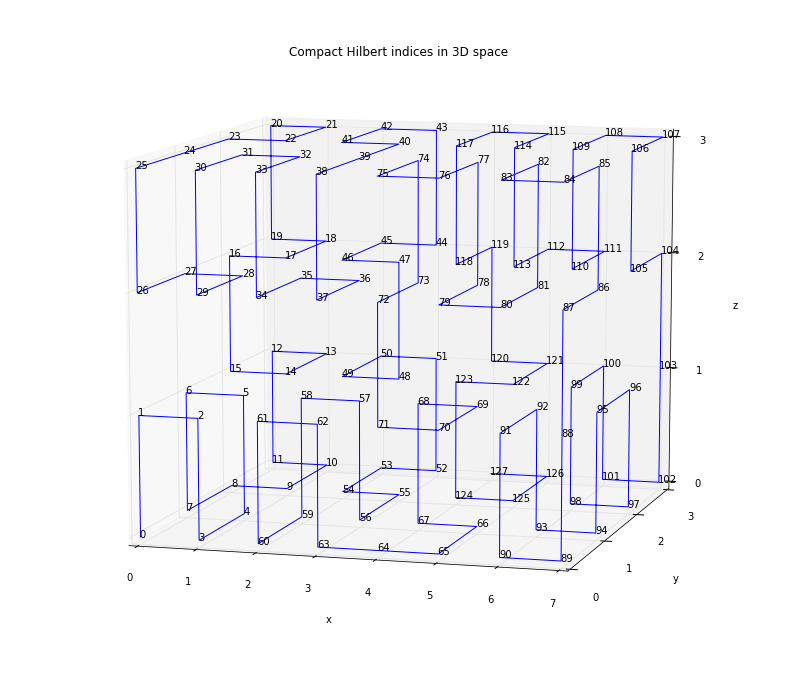In [ ]: